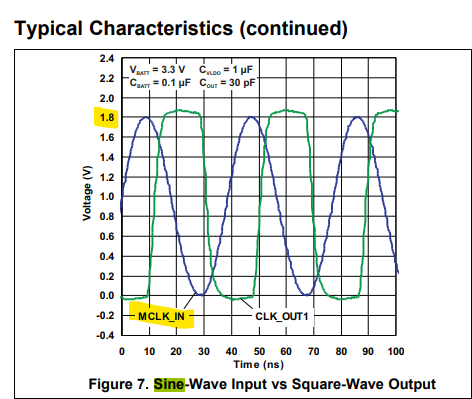If you have a related question, please click the "Ask a related question" button in the top right corner. The newly created question will be automatically linked to this question.

# CDC3RL02: clarification on the value of sine wave input

Part Number: CDC3RL02

Hello Guys,

Good day.

In the datasheet it writes several times that the lowest acceptable sine-wave input is 0.3 Volts, but In the typical characteristics graph it shows the typical sine-wave input swinging from 0V to 1.8VIn the electrical specifications it shows the lowest as being .3V but is that P-P? In the actual typical characteristics on page 8 it shows a sine wave swinging from 0 to 1.8V being used as the MCLK_IN.

Thanks for the confirmation.

Art

• Hi Art,

The input amplitude is defined as P-P in this datasheet. This means that the buffer accepts a minimum amplitude of 0.3V and maximum of 1.8V.

In the Typical Characteristics section, the figure means to show the characteristics of a 1.8V MCLK_IN input (0 to 1.8V). If a 0.3V MCLK_IN input is to be used, then a 0 to 0.3V sinewave would be shown.

Regards,

Jennifer